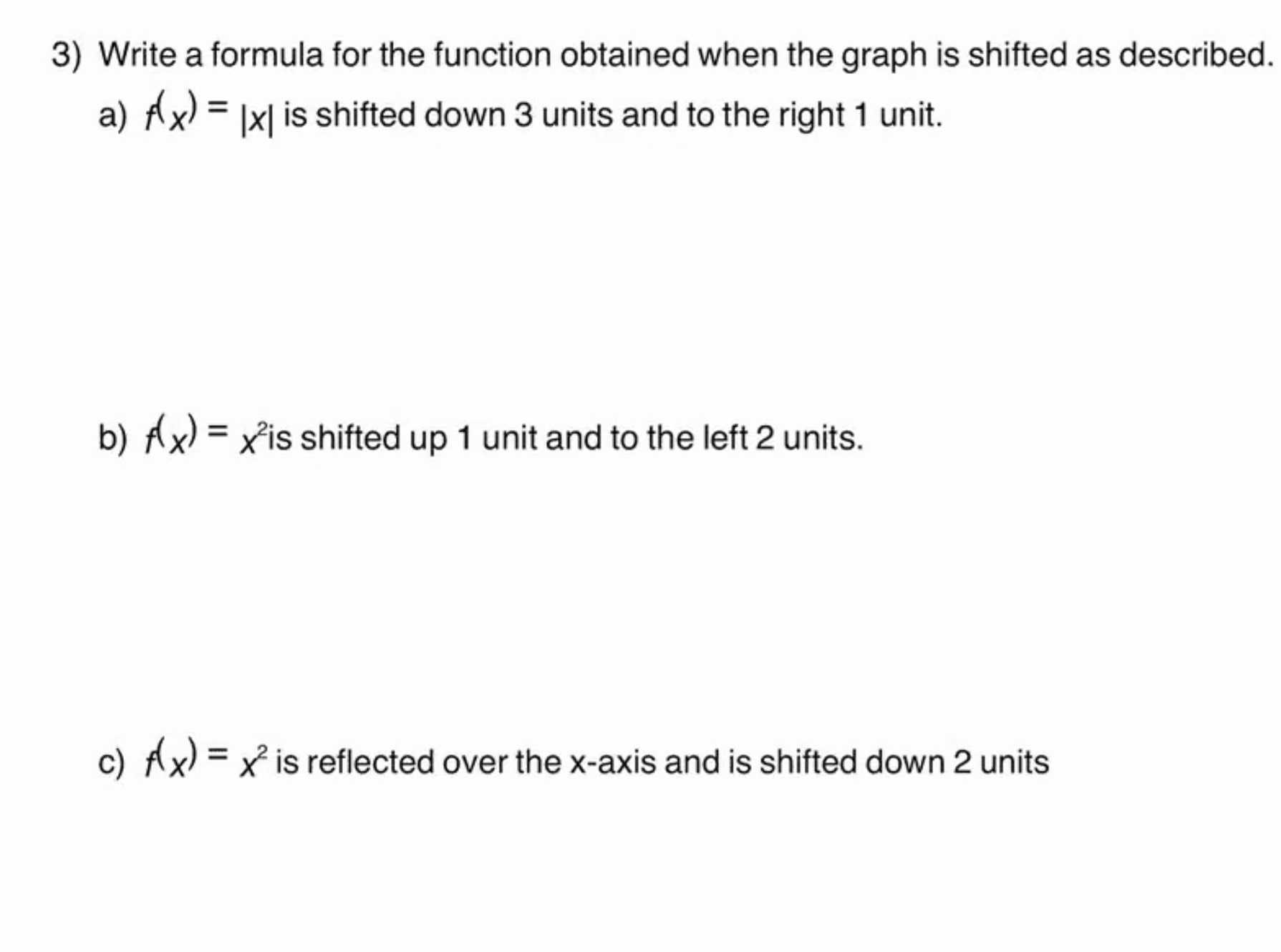### ¿Todavía tienes preguntas de matemáticas?

Pregunte a nuestros tutores expertos
Algebra
Pregunta3) Write a formula for the function obtained when the graph is shifted as described. a) $$f _ { x } ) = | x |$$ is shifted down $$3$$ units and to the right $$1$$ unit. b) $$f _ { x } ) = x ^ { 2 }$$ is shifted up $$1$$ unit and to the left $$2$$ units. c) $$f _ { x } ) = x ^ { 2 }$$ is reflected over the $$x$$ -axis and is shifted down $$2$$ units

a) |x -1| - 3  , b) $$(x+ 2)^2+ 1$$ , c) $$- x^2- 2$$